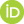# Gödel mathematics versus Hilbert mathematics. II Logicism and Hilbert mathematics, the identification of logic and set theory, and Gödel’s “completeness paper” (1930)

Penchev, Vasil (2023) Gödel mathematics versus Hilbert mathematics. II Logicism and Hilbert mathematics, the identification of logic and set theory, and Gödel’s “completeness paper” (1930). [Preprint]Preview
Text
Gödel mathematics versus Hilbert mathematics.pdf

## Abstract

The previous Part I of the paper (http://philsci-archive.pitt.edu/21280/) discusses the option of the Gödel incompleteness statement (1931: whether “Satz VI” or “Satz X”) to be an axiom due to the pair of the axiom of induction in arithmetic and the axiom of infinity in set theory after interpreting them as logical negations to each other. The present Part II considers the previous Gödel’s paper (1930) (and more precisely, the negation of “Satz VII”, or “the completeness theorem”) as a necessary condition for granting the Gödel incompleteness statement to be a theorem just as the statement itself, to be an axiom. Then, the “completeness paper” can be interpreted as relevant to Hilbert mathematics, according to which mathematics and reality as well as arithmetic and set theory are rather entangled or complementary rather than mathematics to obey reality able only to create models of the latter. According to that, both papers (1930; 1931) can be seen as advocating Russell’s logicism or the intensional propositional logic versus both extensional arithmetic and set theory. Reconstructing history of philosophy, Aristotle’s logic and doctrine can be opposed to those of Plato or the pre-Socratic schools as establishing ontology or intensionality versus extensionality. Husserl’s phenomenology can be analogically realized including and particularly as philosophy of mathematics. One can identify propositional logic and set theory by virtue of Gödel’s completeness theorem (1930: “Satz VII”) and even both and arithmetic in the sense of the “compactness theorem” (1930: “Satz X”) therefore opposing the latter to the “incompleteness paper” (1931). An approach identifying homomorphically propositional logic and set theory as the same structure of Boolean algebra, and arithmetic as the “half” of it in a rigorous construction involving information and its unit of a bit. Propositional logic and set theory are correspondingly identified as the shared zero-order logic of the class of all first-order logics and the class at issue correspondingly. Then, quantum mechanics does not need any quantum logics, but only the relation of propositional logic, set theory, arithmetic, and information: rather a change of the attitude into more mathematical, philosophical, and speculative than physical, empirical and experimental. Hilbert’s epsilon calculus can be situated in the same framework of the relation of propositional logic and the class of all mathematical theories. The horizon of Part III investigating Hilbert mathematics (i.e. according to the Pythagorean viewpoint about the world as mathematical) versus Gödel mathematics (i.e. the usual understanding of mathematics as all mathematical models of the world external to it) is outlined.

 Export/Citation: EndNote | BibTeX | Dublin Core | ASCII/Text Citation (Chicago) | HTML Citation | OpenURL
 Social Networking:

Item Type: Preprint
Creators:
CreatorsEmailORCIDPenchev, Vasilvasildinev@gmail.com0000-0002-9684-8174
Keywords: arithmetic, Aristotle, bit of information, Boolean algebra, first-order logic, Gödel, epsilon calculus, Husserl, logicism, propositional logic, ontology, Pythagoreanism, quantum logic, Russell, set theory
Subjects: Specific Sciences > Mathematics > Logic
General Issues > History of Philosophy of Science
General Issues > History of Science Case Studies
Depositing User: Prof. Vasil Penchev
Date Deposited: 11 Jan 2023 13:29
Item ID: 21639
Official URL: https://www.cambridge.org/engage/coe/article-detai...
DOI or Unique Handle: https://doi.org/10.33774/coe-2023-m1rck
Subjects: Specific Sciences > Mathematics > Logic
General Issues > History of Philosophy of Science
General Issues > History of Science Case Studies
Date: 6 January 2023
URI: http://philsci-archive.pitt.edu/id/eprint/21639

### Monthly Views for the past 3 yearsView Item